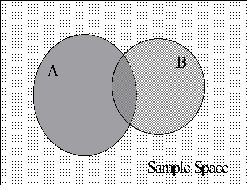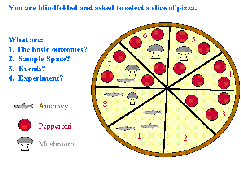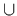# That Was Venn, This Is Now

Bradley A. Warner, David Pendergraft, and Timothy Webb

Journal of Statistics Education v.6, n.1 (1998)

Copyright (c) 1998 by Bradley A. Warner, David Pendergraft, and Timothy Webb, all rights reserved. This text may be freely shared among individuals, but it may not be republished in any medium without express written consent from the authors and advance notification of the editor.

Key Words: Basic probability; Pizza; Venn diagram.

## Abstract

Basic probability concepts are difficult for some students to understand initially. Through the use of a Venn diagram disguised as a pizza, we will discuss how to explain introductory probability concepts. Students are able to answer probability questions, including conditional probability, by simply looking at a picture. This tool not only enhances learning but retention as well.

# 1. Introduction

1 Some students are taught basic probability concepts early in an introductory statistics course. Other students are exposed to these concepts in a course on probability. Unfortunately, many students have no experience with formal probability concepts and find them difficult to comprehend. On the other hand, most students are very familiar with pizza. Capitalizing on this fact, we use a Venn diagram in the guise of a pizza to facilitate the students' understanding of basic probability theory. From this simple tool, ideas such as sample space, outcomes, mutually exclusive events, independence, and conditional probability are easily explained.

# 2. Use of the Venn Pizza Diagram in Class

2 The Venn diagram is a useful tool for graphically representing a sample space. Most Venn diagrams take the form of Figure 1, with a generic rectangle representing the sample space and generic circles representing events obscurely labeled A and B. Although the typical Venn diagram illustrates the basic principles of probability, some students have difficulty visualizing the meaning of the elements of the diagram and then relating them to concepts such as independence. Part of the problem may stem from the abstract presentation of the typical Venn diagram. Believing in the value of the Venn diagram as an important tool in learning basic probability, we have adopted a Venn diagram that takes the form of a pizza, Figure 2. This representation is more concrete for students and allows them to intuitively understand the basic probability concepts.Figure 1 (10.5K gif)

Figure 1. Typical Venn Diagram.Figure 2 (14.8K gif)

Figure 2. Venn Diagram in the Form of a Pizza.

3 In Figure 2, the pizza represents the sample space. Different events are portrayed by the toppings on the pizza. As a quick example of the power of this representation, show someone with no formal probability education Figure 2 and ask him or her, "You have pulled out a slice of pizza that contains pepperoni. What is the probability that it has mushrooms?" Most people correctly answer 3/5, even if they have had no exposure to conditional probabilities.

4 In our introductory probability lecture, we require the students to understand the basic notation, terms, and properties of probability. To achieve this objective, we use Figure 2 in a question and discussion format. The students typically answer the questions correctly while the discussion allows the instructor to relate the questions back to probability concepts. The basic probability concepts are easily covered in one or two lessons using the Venn pizza. The usual questions about events and their intersections, unions, and compliments are easily translated into the pizza context. For example:

• "What is the probability that your slice will have pepperoni or mushrooms?"

• "Given that your slice has anchovies, what is the probability that it will have mushrooms?"

5 We have found the use of the Venn pizza to be particularly helpful to students in understanding the concepts of a basic outcome, conditional probability, statistical independence, and why the calculation of the union requires the subtraction of the intersection. In a typical Venn diagram, the student is told that a randomly selected point in the rectangle represents a basic outcome. However, since they can not see this point, it is difficult for them to intuitively understand a basic outcome. With the Venn pizza, the student understands the concept of a slice of pizza and thus has a physical sense of a basic outcome.

6 Conditional probability and statistical independence are arguably the most difficult concepts for students to understand. Conditional probability and independence are difficult to visualize in a traditional Venn diagram because the probabilities are represented by areas. In this scenario, we are counting on the students to not only judge the size of areas, but to judge a ratio of the size of areas. It is our experience that many students are not adept at this task. In the Venn pizza, the students immediately restrict the sample space based on the conditioning event. When told that "Your slice has pepperoni," the student instinctively uses a denominator of five for the conditional probability calculations. Independence can also be easily illustrated by the Venn pizza. Figure 3 demonstrates a Venn diagram where the events pepperoni and mushroom are independent. Figure 3 used in conjunction with Figure 2, where pepperoni and mushroom are not independent, illustrates the concept of independence well.Figure 3 (11.9K gif)

Figure 3. Venn Diagram with Independent Events.

7 Another potentially troubling concept for some students is why the joint probability of two events must be subtracted out when determining the union of these two events. With the Venn pizza, it becomes clear that when the probabilities of the individual events are summed, the slices with both toppings are added in twice.

# 3. Discussion

8 The Venn pizza diagram is an excellent way to introduce the concepts and properties of probability. However, after presenting the lecture, you may want to stretch the students by presenting more difficult problems. Some example questions are:

1. What is the P(AnchovyMushroomPepperoni)?

2. Given that a single pizza topping selected off a slice of pizza is pepperoni, what is the probability it came from slice number 3?

3. You select two slices of pizza. What is the probability the second slice has pepperoni?

4. Using the statistically independent pizza (Figure 3), are the events MushroomC and Pepperoni independent? What generalization about independent events can you make?

5. Suppose the probability of selecting a slice of pizza is proportional to the size of the slice. In Figure 2, all the slices are the same size. Now combine slices 4 and 5 into one slice, which is now twice as big as the other slices. Using this new modified pizza, what is the probability of pepperoni on your slice of pizza given that you selected a slice with mushrooms?

9 Of course, these questions are testing advanced concepts such as Bayes' Rule, sampling without replacement, averaging conditional probabilities, and determining the union of more than two events. The last question is designed to help students realize that the initial probability calculations were based on the assumption of equally likely outcomes. This question causes them to investigate what happens when that assumption does not hold. Another way to present this same concept is to draw a new pizza in which every slice is a different size. The problem with this approach is that the students are expected to estimate areas, a point we were critical of in the traditional Venn diagram.

10 Over the course of the semester, the basic probability concepts will come up again. Unfortunately, a high percentage of students forget what they learned in the introductory probability lesson, but by showing them Figure 2, the concepts come back quickly. If used often enough, the students realize that if they ask a basic probability question, they will see the Venn pizza. They soon use the Venn pizza to answer their own questions.